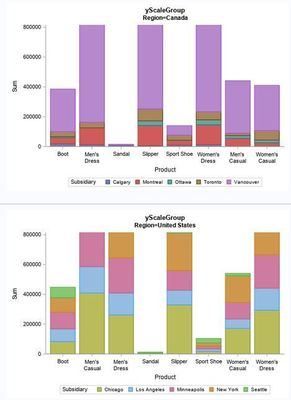## proc sgplot, vbarbasic and uniform=yscalegroup (truncated y-axis)

Hi,

I'm trying to use uniform=yscalegroup with vbarbasic.

I'm not sure why the y-axis get truncated at \$800,000 unless the maximum values is specified explicitly in a yaxis statement.

The y-axis is not trucated when using uniform=group but the y-axis are not harmonized.

I agree that uniform=group is the more likely scenario

but it would nice to understand where the issue comes from.

Having said that, there is this sentence in the SAS online documentation I don't fully understand (lost in translation):

"Basic bar charts (HBARBASIC and VBARBASIC) do not support uniform axis scaling. However, they do support group uniformity."

Could it be the reason?

``````proc sort data=shoes (where=(region in ('Canada','United States'))) out=shoes;
by region subsidiary product;
run;

ods exclude summary;
ods output summary=shoes_sum;
proc means data=shoes sum;
by region subsidiary product;
var sales;
run;

ods graphics / width=15cm height=10cm noborder;

title 'yScaleGroup';
proc sgplot data=shoes_sum noborder uniform=yscalegroup;
by region;
vbarbasic product / response=sales_sum
group=subsidiary;
*yaxis values=(0 to 2200000 by 200000);
run;

ods graphics off;``````1 ACCEPTED SOLUTION

Accepted SolutionsDanH_sas
SAS Super FREQ

## Re: proc sgplot, vbarbasic and uniform=yscalegroup (truncated y-axis)

The real issue with VBARBASIC/HBARBASIC is that uniform "response" axes are not supported. Therefore UNIFORM=ALL and UNIFORM=SCALE should *NEVER* be used with these statements. UNIFORM=GROUP has no effect on axes, so this option is okay. UNIFORM=XSCALE | XSCALEGROUP should work for VBARBASIC. and UNIFORM=YSCALE | YSCALEGROUP should work for HBARBASIC, as you will affecting only the category axis.

That being said, I believe you can also use the VALUESLIST option to control the list and order of your category values, but you would need to know the list ahead of time. Again, given your need for uniformity, I would recommend that you consider using VBAR/HBAR or VBARPARM/HBARPARM so that all UNIFORM options are available to you without these special considerations.

5 REPLIES 5

## Re: proc sgplot, vbarbasic and uniform=yscalegroup (truncated y-axis)

try this:

``````ods select summary;
ods listing;``````

## Re: proc sgplot, vbarbasic and uniform=yscalegroup (truncated y-axis)

Hi, The issue is not on the proc means but on the proc sgplot.DanH_sas
SAS Super FREQ

## Re: proc sgplot, vbarbasic and uniform=yscalegroup (truncated y-axis)

That statement is correct -- uniform axis scaling is not available when using VBARBASIC or HBARBASIC. However, there is nothing you can do with VBARBASIC and HBARBASIC that you can't do by using VBAR/HBAR or VBARPARM/HBARPARM (depending on the situation). The VBARBASIC and HBARBASIC statements are convenience statements for when you want to specify a basic statistic (sum | mean | freq) on a bar chart *AND* overlay one or more "basic" plot types (scatter, series, etc.).

In general,VBAR/HBAR statements are your best choice when you want to used the statistics available in the procedure and you have only VLINE/HLINE, DOT, or other VBAR/HBAR overlays (or no overlays). If you do have a need to overlay other plot types, use VBARPARM/HBARPARM and precompute your statistics using PROC SUMMARY or PROC MEANS. Each of these four bar chart statements support uniform axis scaling.

## Re: proc sgplot, vbarbasic and uniform=yscalegroup (truncated y-axis)

Thanks @DanH_sas

So to make sure I properly understand the statement

"Basic bar charts (HBARBASIC and VBARBASIC) do not support uniform axis scaling. However, they do support group uniformity"

1/ "group uniformity" would mean that values of the variable listed in the group= option can be harmonized across graphs generated with the by statement
i.e. the legend can be common to all graphs when using uniform=group, xscalegroup or yscalegroup.

The harmonizsation of the values of the category variable is not foreseen with those options.
However in my test, uniform=xscalegroup harmonizes the values of the category variable when displayed on the x-axis (vbarbasic)
and uniform=yscalegroup harmonizes the values of the category variable displayed on the y-axis (hbarbasic)
but uniform=group does not harmonize the values of the category variable.

By default, the legend is specific to each graph.
According to the SAS Online Documentation uniform=all by default.
But when I use it, the y-axis gets truncated. (I tried all the values listed in the documentation).

2/ "do not support uniform axis scaling" means that uniform=scale, xscale, yscale, all would have no effect when using vbarbasic or hbarbasic.

However, when there is no group variable in the statement, I could see in my test that xscale/yscale and xscalegroup/yscale affects the order of the values of the category variable.

Only uniform=group does not affect the order of the values of the category variables (as in the default setting).
The other values (all, scale) would truncate the response axis.

-----

So if group= is used, we should consider using uniform=group to harmonize the legend but should prefer another method to set the order of the values of the category variable.
We should not use the default group=all explicitely to avoid response axis truncation.

If no group= is used, we should not use the uniform= option and we should use another method to set the order of the values of the category variable.

Correct?DanH_sas
SAS Super FREQ

## Re: proc sgplot, vbarbasic and uniform=yscalegroup (truncated y-axis)

The real issue with VBARBASIC/HBARBASIC is that uniform "response" axes are not supported. Therefore UNIFORM=ALL and UNIFORM=SCALE should *NEVER* be used with these statements. UNIFORM=GROUP has no effect on axes, so this option is okay. UNIFORM=XSCALE | XSCALEGROUP should work for VBARBASIC. and UNIFORM=YSCALE | YSCALEGROUP should work for HBARBASIC, as you will affecting only the category axis.

That being said, I believe you can also use the VALUESLIST option to control the list and order of your category values, but you would need to know the list ahead of time. Again, given your need for uniformity, I would recommend that you consider using VBAR/HBAR or VBARPARM/HBARPARM so that all UNIFORM options are available to you without these special considerations.

Discussion stats
• 5 replies
• 351 views
• 2 likes
• 3 in conversation